Keldysh theorem

Keldysh' theorem on approximating continuous functions by polynomials. Letbe a function of a complex variablethat is holomorphic in a domainand continuous in the closed domain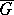. Then in order that for anya polynomial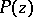exists such that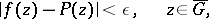it is necessary and sufficient that the complement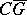consists of a single domain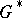containing the point at infinity. The theorem was established by M.V. Keldysh . It is one of the basic results in the theory of uniform approximation of functions by polynomials in the complex domain (see ).

Keldysh' theorems in potential theory are theorems on the solvability of the Dirichlet problem, established by M.V. Keldysh in 1938–1941.

a) Letbe a bounded domain in the Euclidean space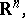, with boundary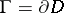. Then there exists ona countable set of irregular boundary points (cf. Irregular boundary point)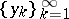, such that the Dirichlet problem is solvable inwith a continuous boundary functiononif and only if this problem is solvable at,that is, if and only ifwhere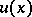is the generalized solution of the Dirichlet problem in the sense of Wiener–Perron (see Perron method, and also , ).

b) Letbe an operator acting from the space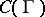of continuous functions oninto the space of bounded harmonic functions (cf. Harmonic function) inand satisfying the following conditions:),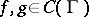, whereare real numbers; that is,is linear;) if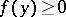,, then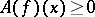; and) if the Dirichlet problem is solvable for an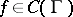, then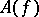gives the solution of this problem. Under these conditionsis unique for, andgives a generalized solution of the Dirichlet problem in the sense of Wiener–Perron (see ).

c) In order that each solvable Dirichlet problem inbe stable in, it is necessary and sufficient that the set of irregular boundary points of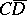coincide with the set of irregular boundary points of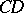. The Dirichlet problem is stable in the interior ofwith respect to any functionif and only if the set of irregular boundary points ofbelonging tohas zero harmonic measure in(see  or ).